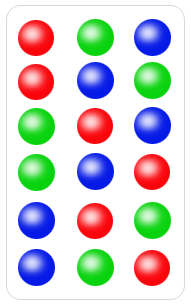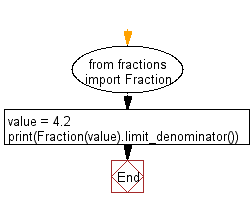﻿ Python Math: Generate all permutations of a list in Python - w3resource# Python Math: Generate all permutations of a list in Python

## Python Math: Exercise-24 with Solution

Write a Python program to generate all permutations of a list in Python.

In mathematics, the notion of permutation relates to the act of arranging all the members of a set into some sequence or order, or if the set is already ordered, rearranging (reordering) its elements, a process called permuting. These differ from combinations, which are selections of some members of a set where order is disregarded.

In the following image each of the six rows is a different permutation of three distinct ballsSample Solution:-

Python Code:

``````import itertools
print(list(itertools.permutations([1,2,3])))
```
```

Sample Output:

```[(1, 2, 3), (1, 3, 2), (2, 1, 3), (2, 3, 1), (3, 1, 2), (3, 2, 1)]
```

Flowchart:## Visualize Python code execution:

The following tool visualize what the computer is doing step-by-step as it executes the said program:

Python Code Editor:

Have another way to solve this solution? Contribute your code (and comments) through Disqus.

What is the difficulty level of this exercise?

Test your Python skills with w3resource's quiz

﻿

## Python: Tips of the Day

Python: Get the Key Whose Value Is Maximal in a Dictionary

```>>> model_scores = {'model_a': 100, 'model_z': 198, 'model_t': 150}
>>> # workaround
>>> keys, values = list(model_scores.keys()), list(model_scores.values())
>>> keys[values.index(max(values))]
'model_z'
>>> # one-line
>>> max(model_scores, key=model_scores.get)
'model_z'
```